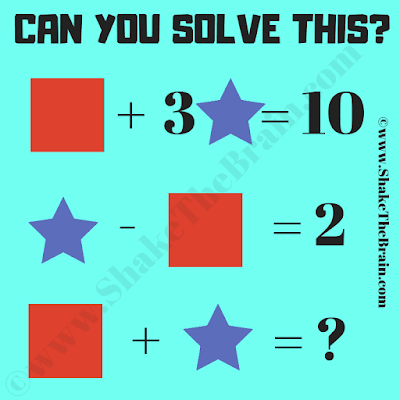Prepare to embark on an exhilarating mathematical journey with the captivating "Maths Shapes Algebra Problem: Solve Equations and Discover." This puzzle beckons you to explore the intriguing realm of algebraic equations intertwined with geometric shapes, inviting you to flex your analytical muscles and decipher the hidden relationships within.

The puzzle presents an array of intricate algebraic equations, each featuring various mathematical shapes with values assigned to them. Your task? To unravel the numerical values that correspond to each shape, unravel the mathematically encoded clues concealed within the equations. As you meticulously work your way through the equations, you'll be unlocking the secrets that bridge the gap between shapes and their values.Can you find the value of the missing number?

Yet, the maths puzzle challenge doesn't conclude there. The puzzle's pinnacle lies in a final equation, adorned with a missing number. Armed with the insights you've gleaned from solving the equations, your ultimate quest is to decipher the enigma of the missing number. This requires not only a keen understanding of algebraic relationships but also the ability to perceive patterns and connections that lie beneath the surface.

The "Maths Shapes Algebra Problem" is an exquisite fusion of mathematical deduction and logical reasoning, designed to ignite your cognitive faculties and immerse you in the world of mathematical exploration. Whether you revel in the complexities of algebra or find joy in the pursuit of logical solutions, this puzzle offers an immersive experience that simultaneously challenges and rewards your intellect.

As you unravel the layers of the equations, you'll find yourself deciphering the language of algebraic expressions, making insightful deductions, and experiencing the thrill of cracking intricate puzzles. Yet, this puzzle isn't just about finding answers—it's about engaging in a quest for understanding, where each equation contributes a piece to the larger puzzle, leading you ever closer to unveiling the mystery of the missing number.

So, are you ready to embark on this mathematical odyssey? Can you decipher the connections between shapes, uncover the values, solve equations, and ultimately unveil the missing number's hidden identity? Prepare to embark on a journey that promises both mental stimulation and the gratification of unraveling the complex web of mathematical intrigue.

The answer to this "Maths Shapes Equations Picture Puzzle", can be viewed by clicking on the answer button.

#### 1 comment:

Unknown said...

There are two answers. The one you have here but also the answer could be 6 with Star = 2 and Square = 4.